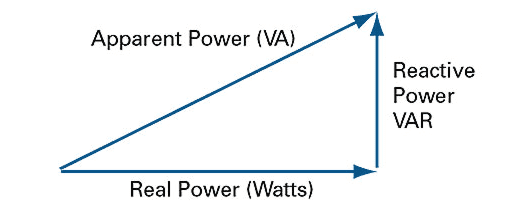# What is "Apparent Power" (VA)?

iScience
In school I learned about impedance:
where..

$$Z^2 = R^2 + X_{net}^2$$

but this was the only triangle I recall learning about.
The picture below I definitely do not recall.The only quantity I am familiar with is the x-axis (Power).

If someone could explain apparent power and its importance with finding an appropriate UPS device, please throw me a bone. ty

•berkeman

•DaveE
Staff Emeritus
Read that article that @gleem posted for you.

For a dead simple example, a 100 VA UPS could power a 100 w resistance heater, but not a 100 w motor. That's why it is important.

In a resistance, the imaginary power is zero, so VA and power are the same thing. That's what you are used to.

The motor needs both real power (watts) and imaginary power, so the VA is the complex sum as shown in the diagram you posted in #1.

••sophiecentaur and berkeman